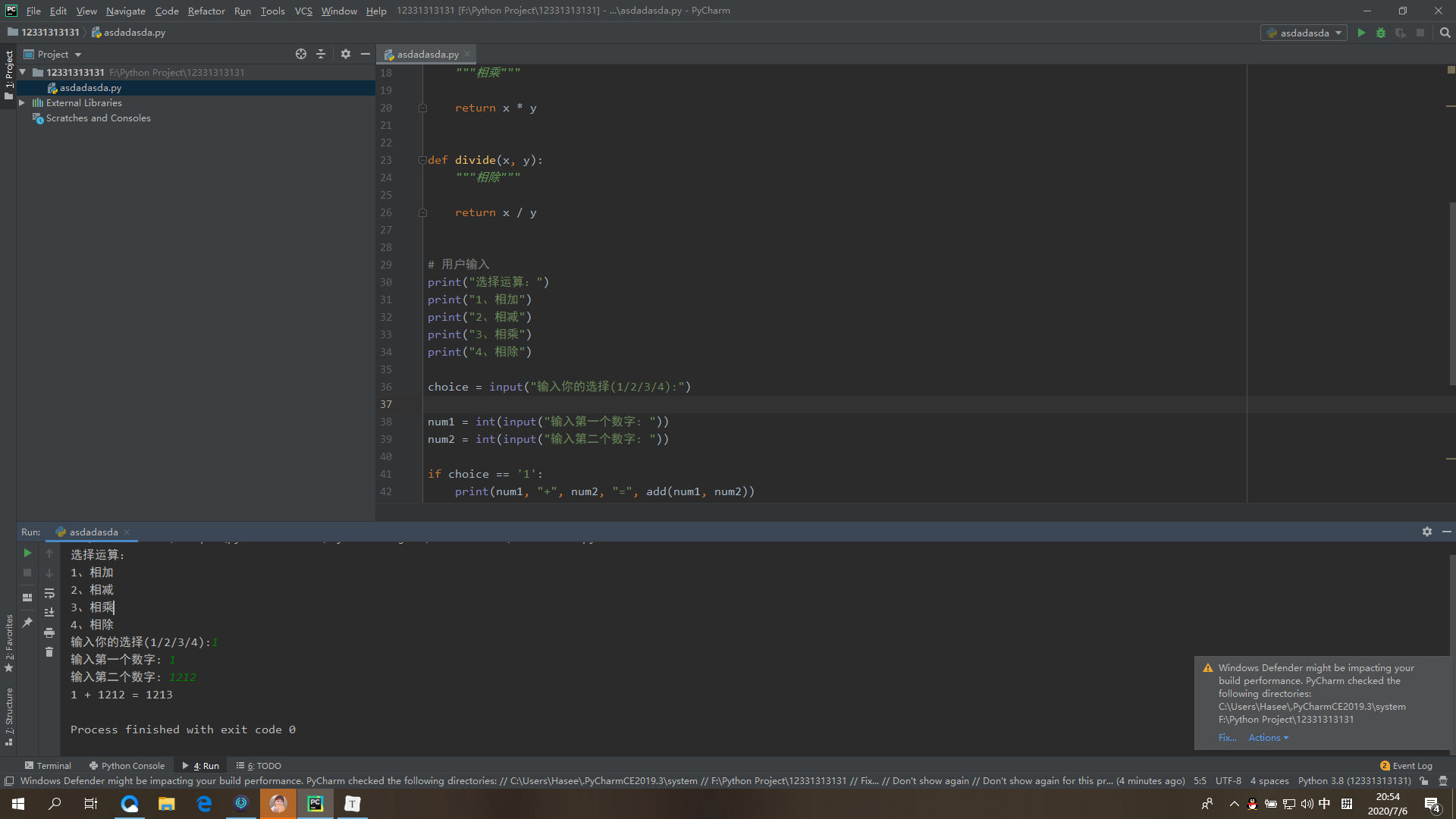# 学号 20193103陈柏维《Python程序设计》实验二报告

## 1.实验内容

• 设计并完成一个完整的应用程序，完成加减乘除模等运算，功能多多益善

• 考核基本语法、判定语句、循环语句、逻辑运算等知识点

## 2. 实验过程及结果

# Filename : test.py# author by : www.runoob.com # 定义函数def add(x, y):   """相加"""    return x + y def subtract(x, y):   """相减"""    return x - y def multiply(x, y):   """相乘"""    return x * y def divide(x, y):   """相除"""    return x / y # 用户输入print("选择运算：")print("1、相加")print("2、相减")print("3、相乘")print("4、相除") choice = input("输入你的选择(1/2/3/4):") num1 = int(input("输入第一个数字: "))num2 = int(input("输入第二个数字: ")) if choice == '1':   print(num1,"+",num2,"=", add(num1,num2)) elif choice == '2':   print(num1,"-",num2,"=", subtract(num1,num2)) elif choice == '3':   print(num1,"*",num2,"=", multiply(num1,num2)) elif choice == '4':   print(num1,"/",num2,"=", divide(num1,num2))else:   print("非法输入")## 3. 实验过程中遇到的问题和解决过程

• 问题一:考虑如何使程序进行多次计算

• 问题一解决方案：通过循环多次进行操作

## 其他（感悟、思考等）

python程序的各种函数有无数种组合方式，每次组合都会造就不同的结果。

## 参考资料

• [《Java程序设计与数据结构教程（第二版）》]

• [《Java程序设计与数据结构教程（第二版）》学习指导]

posted @ 2020-07-06 20:54  陈柏维  阅读(82)  评论(0编辑  收藏